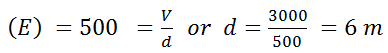# The formation of a dipole is due to two equal and dissimilar point charges placed at a (a)    Short distance (b)    Long distance (c)    Above each other (d)    None of these

9 years ago

(a)

Dipole is formed when two equal and unlike charges are placed at a short distance.

9 years ago

(a)

Given: Electric field (E) = 500 V/m and potential difference

(V) = 3000 V.

We know that electric field[where d = Distance between point charge and A]